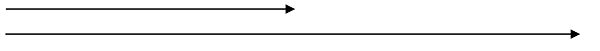• 标量与向量
千次阅读
2021-04-27 20:58:38

## 标量与向量

1、用维数来定义标量与向量，一维的量称之为标量，超过一维的量称之为向量，所以有二维向量，三维向量，以及n维向量。每一维表示量的一种独立特征，维数越高对量的表示越精确坐标表示量在维上的投影（投影有正负，这与本维规定的单位向量方向有关），坐标表示量在维上的分量，坐标表示量的独立特征所以用坐标表示量维上规定的单位向量称为基，由于不同维表示量的不同独立特征，所以不同基相互独立（正交）。所以用两位坐标（x,y）表示二维向量，三位坐标（x,y,z）表示三维向量，用n位坐标（x1,x2,…,xn）表示n维向量。
2、平面向量是二维向量，用两位坐标表示，比如（x,y），x坐标表示量在本维的投影，表示量在本维的分量，表示量的一种独立特征。 空间向量是三维向量，用三维坐标表示，比如（x,y,z）。n维向量用n位坐标表示（x1,x2,…,xn），每位坐标表示量在本维的投影，表示量在本维的分量，表示量的一种独立特征。

## 标量、向量与数

标量用实数表示，二维向量用复数表示。
二维向量也可用平面有向线段表示，三维向量也可用空间有向线段表示。

更多相关内容
• a=np.array([True,True,False,False]) #当为布尔类型，做或非操作 b=np.array([True,False,True,False]) c=np.array([1,2,3]) d=np.array([2,1,2]) print (a|b) #布尔或 print (a&b) #布尔 print (~a) #布尔非 ...

# 1、简单运算

+、-、*、/、**：加减乘除幂运算

import numpy as np
a=np.array([1,2,3])
b=np.array([3,2,1])
print (a+10) #对应位置依次相加
print (a*2)
print ([1,2,3]*2)
print (a+b)
print (a-b)
print (a*b)
print (a/b)
print (a**b) #次幂操作
[11 12 13]
[2 4 6]
[1, 2, 3, 1, 2, 3]
[4 4 4]
[-2  0  2]
[3 4 3]
[0.33333333 1.         3.        ]
[1 4 3] 

# 2、布尔运算

&、|、~：与或非运算

• 当为boolean时，是与或非
• 当为整数时，按位做与或非操作

如3&2，3按照二进制为11，2按二进制为10，3&2就相当于11&10，得到了10为2

3&2=2

3|2=3

非操作，如~3，，机器是32位了，3=0000......0000 0011,非操作就是把所有的0变成1，1变成0，

~3=1111......1111 1100,计算机中首位是1表示一个负数，后面的数用补码实现，一般是把补码取非操作，然后再+1得到自然数

即：1后面的很多0取非操作，0000......0000 0011=3,3+1=4,再加一个符号为-4

所以~3=-4

~n=-(n+1)

import numpy as np
a=np.array([True,True,False,False]) #当为布尔类型，做与或非操作
b=np.array([True,False,True,False])
c=np.array([1,2,3])
d=np.array([2,1,2])
print (a|b) #布尔或
print (a&b) #布尔与
print (~a)  #布尔非
print (c|d) #按位或
print (c&d) #按位与
print (~c)  #按位非
[ True  True  True False]
[ True False False False]
[False False  True  True]
[3 3 3]
[0 0 2]
[-2 -3 -4]

# 3、比较运算

>、>=、<、<=、==、!=大于小于等于不等运算

import numpy as np
a=np.array([1,2,3])
b=np.array([3,2,1])
print (a>b)
print (a>=b)
print (a<a)
print (a<=b)
print (a==b)
print (a!=b)  
[False False  True]
[False  True  True]
[False False False]
[ True  True False]
[False  True False]
[ True False  True]

展开全文class Vecter3:

def_init_(self,x=0,y=0,z=0):

self.X=x

self.Y=y

self.Z=z

r=Vecter3()

r.X=self.X+n.X

r.Y=self.Y+n.Y

r.Z=self.Z+n.Z

return r

def_sub_(self,n):

r=Vecter3()

r.X=self.X-n.X

r.Y=self.Y-n.Y

r.Z=self.Z-n.Z

return r

def_mul_(self,n):

r=Vecter3()

r.X=self.X*n

r.Y=self.Y*n

r.Z=self.Z*n

return r

def_truediv_(self,n):

r=Vecter3()

r.X=self.X/n

r.Y=self.Y/n

r.Z=self.Z/n

return r

def_floordiv_(self,n):

r=Vecter3()

r.X=self.X//n

r.Y=self.Y//n

r.Z=self.Z//n

return r

def show(self):

print(self.X,self.Y,self.Z))

展开全文• 线性代数向量乘法Prerequisite: Linear Algebra | Defining a Vector 先决条件： 线性代数| 定义向量 Linear algebra is the branch of mathematics concerning linear equations by using vector spaces and ...

线性代数向量乘法

Prerequisite: Linear Algebra | Defining a Vector

先决条件： 线性代数| 定义向量

Linear algebra is the branch of mathematics concerning linear equations by using vector spaces and through matrices. In other words, a vector is a matrix in n-dimensional space with only one column. In linear algebra, there are two types of multiplication:

线性代数是使用向量空间和矩阵的线性方程组的数学分支。 换句话说，向量是n维空间中只有一列的矩阵。 在线性代数中，有两种类型的乘法：

1. Scalar Multiplication

标量乘法

2. Cross Multiplication

交叉乘法

In a scalar product, each component of the vector is multiplied by the same a scalar value. As a result, the vector’s length is increased by scalar value.

在标量积中，向量的每个分量都乘以相同的标量值。 结果，向量的长度增加了标量值。

For example: Let a vector a = [4, 9, 7], this is a 3 dimensional vector (x,y and z)

例如：令向量a = [4、9、7]，这是3维向量(x，y和z)

So, a scalar product will be given as b = c*a

因此，标量积将给出为b = c * a

Where c is a constant scalar value (from the set of all real numbers R). The length vector b is c times the length of vector a.

其中c是常数标量值(来自所有实数R的集合)。 长度矢量b是向量a的长度c倍。## 向量标量乘法的Python代码 (Python code for Scalar Multiplication of Vector)

# Vectors in Linear Algebra Sequnce (5)
# Scalar Multiplication of Vector

def scalar(c, a):
b = []
for i in range(len(a)):
b.append(c*a[i])
return b

a = [3, 5, -5, 8] # This is a 4 dimensional vector

print("Vector a = ", a)
c = int(input("Enter the value of scalar multiplier: "))

# The vector b will have the same dimensions
# but the overall magnitute is c times a
print("Vector (b = c*a) = ", scalar(c, a))



Output

输出量

Vector a =  [3, 5, -5, 8]
Enter the value of scalar multiplier: 3
Vector (b = c*a) =  [9, 15, -15, 24]




线性代数向量乘法

展开全文python 机器学习 神经网络 算法
• Python 3.4.2代码如下 class Vecter3:def __init__(self, x=0, y=0, z=0):self.X = xself.Y = yself.Z = zdef __add__(self, n):r = Vecter3()r.X = self.X + n.Xr.Y = self.Y + n.Yr.Z = self.Z + n.Zreturn rdef _...
• ## 张量、向量、标量的区别

万次阅读 多人点赞 2018-04-26 14:14:53
标量是0阶张量(一个数)，是1*1的； 向量是一阶张量，是1*n的； 张量可以给出所有坐标间的关系，是n*n的。 所以通常有人说将张量(n*n)reshape成向量(1*n)，其实reshape过程中并没有发生大的变化。 参考地址:...
• 在定义向量线网时可选用关键词scalared 或vectored。如果一个线网定义时使用了关键词vectored, 那么就不允许位选择和部分选择该线网。换句话说，必须对线网整体赋值（位选择和部分选择在下一章中讲解）。例如:wire ...
• 矩阵、向量都可以表示成张量的形式，向量是矩阵的特殊形式，按实际应用可分为标量向量求导，标量对矩阵求导、向量向量求导、矩阵对标量求导、矩阵对向量求导、矩阵对矩阵求导等，在深度学习的反向传播(BP)中所...机器学习 深度学习
• numpy 向量乘法Prerequisite: Defining Vector using Numpy 先决条件： 使用Numpy定义Vector To read about the Scalar Multiplication of Vector, ... 要了解向量标量乘法，请访问： 向量标量乘法 Here, we...python numpy 机器学习 深度学习
• 这个 m 文件可以返回以度数或两个向量标量积的 dms 系统缩放的角度。 两个向量的长度必须相同。 语法：function andop(A,B,s) 输入： A 和 B - 大小相等的向量。 s - 以度数 [=1（默认）] 或 dms 系统 [~=1] 为...matlab
• 1 class vector3:2 def __init__(self, x_ = 0, y_ = 0, z_ = 0):3 self.x = x_4 self.y = y_5 self.z = z_6 def __add__(self, obj):7 return vector3(self.x+obj.x, self.y+obj.y, self.z+obj.z)8 def ...
• 数据类型numpy和tensor 当pytorch提出数据类型不对的时候，可以查看数据类型以及修改成正确的类型。废话少说：直接上代码 train_x,test_x,train_y,test_y=train_test_split(X,Y) print(type(train_x)) ...pytorch 深度学习 python
• 1.已知    对谁求导数，就以谁...关于x的转置x.T求导数，x.T是行向量，那么Ax分别对x.T向量中的分量求偏导（写成一列），然后整体排成一行（同x.T是行向量）。 2.若A是1×n行向量，x是n×1的列向量， 有  3...
• 标量向量，矩阵张量 1、标量 一个标量就是一个单独的数，一般用小写的的变量名称表示。   2、向量 一个向量就是一列数，这些数是有序排列的。用过次序中的索引，我们可以确定每个单独的数。通常会赋予向量...
• https://www.cnblogs.com/pinard/p/10773942.html
• 计算机中的标量机是指只是一个数一个数地进行计算的加工处理方法，区别向量机能够对一批数据同时进行加工处理。标量机比向量机的运算速度慢，因此，向量机更适合于演算数据量多的大型科学、工程计算问题。计算机...
•CMake
• 关于x的转置x.T求导数，x.T是行向量，那么Ax分别对x.T向量中的分量求偏导（写成一列），然后整体排成一行（同x.T是行向量）。 2.若A是1×n行向量，x是n×1的列向量， 有 3.若A是n×1列向量，x是n×1的列向量，有 4...
• 向量X转换成标量，且向量X只能为一维含单个元素的向量 X：nd类型的数据 创建一个单个元素的向量 test=nd.array() 输出： [2.] <NDArray 1 @cpu(0)> 转换成标量： test.asscalar()...mxnet
• 本文实例讲述了Python计算三维矢量幅度的方法。分享给大家供大家参考。具体如下： from numpy import * from math import * a=(['x','y','z']) ...for i in range(3): y=input("Enter %s component:"%a[i]) ...
• MATLAB变量——标量向量，矩阵MATLAB以NM×的形式保存了大量的矩阵，其中M是行数，N是列数。一个1×1矩阵是一个标量；1×N矩阵是一个行向量，，M×1矩阵是一个列向量。矩阵的全部元素可以是实数，也可以是复数；...
• 标量的运算 标量与标量 import torch x = torch.tensor([3.0]) y = torch.tensor([2.0]) print(x + y) print(x * y) print(x / y) print(x ** y) 标量与向量pytorch python 深度学习...